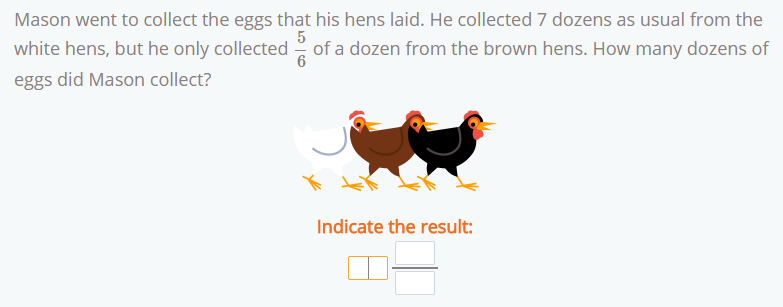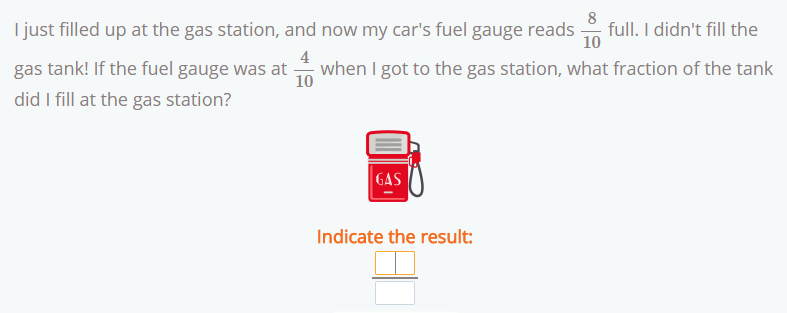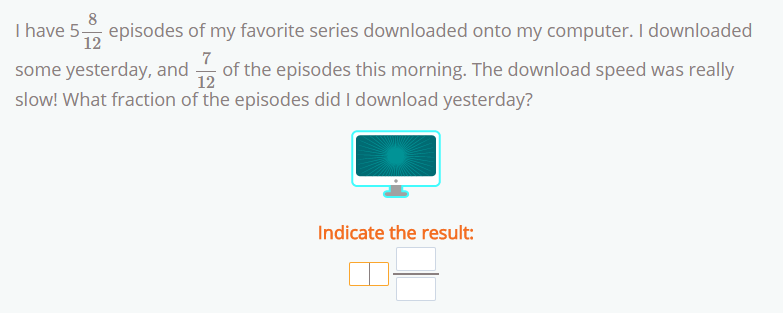Smartick is an advanced online program that teaches kids math and coding in only 15 min. a day

Oct04

# Fraction Word Problems: Addition, Subtraction, and Mixed Numbers

In today’s post, we’re going to see how to solve some of the new problems that we’ve introduced in Smartick, fraction word problems. They appear during the word problems at the end of the daily session.

We’re going to look at how to solve problems involving addition and subtraction of fractions, including mixed fractions (the ones that are made up of a whole number and a fraction).

Try and solve the fraction word problems by yourself first, before you look for the solutions and their respective explanations below.

#### Fraction word problems nº 1#### Fraction word problems nº 2#### Fraction word problems nº 3#### Solution to Problem nº 1:

This is an example of a problem involving the addition of a whole number and a fraction.

In this problem, the simplest way to show how many dozens there are is by using a mixed number, since the result is practically presented to us by the data. 7 dozens + 5/6 of a dozen = 7 5/6 dozens.

#### Solution to Problem nº 2:

In this example, we have to subtract two fractions with the same denominator.

To calculate how full the gas tank is, we have to subtract both fractions. Since we are presented with fractions, the best way to give the solution is in the form of a fraction. Since we’re dealing with two fractions with the same denominator, we just have to subtract the numerators of both fractions to get the result. 8/104/10 = 4/10

#### Solution to Problem nº 3:

This problem requires us to subtract a mixed number and a fraction.

To solve this problem, we need to subtract the number of episodes that were downloaded this morning from the number of episodes that are now downloaded in total. To do this, we need to change the mixed number into a fraction: the 5 becomes 60/12 (5 x 12 = 60) and we add it to the fraction 60/12 + 8/12 = 68/12. We’ve converted the mixed number 5 8/12 to 68/12. Now we just have to subtract the number of episodes that were downloaded yesterday (7/12),  68/127/12 = 61/12.

Hopefully, you didn’t need the explanations and were able to solve them yourself without any help!

If you want to practice with more fraction word problems like these and others, log in to Smartick and enjoy learning math.